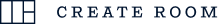### Mini Cart

• No products in the cart.
DISCOVER WHAT MAKES THE NEW DREAMBOX EVEN BETTER LEARN MORESold out

# Crafter's Caddy

Description/Details

Out of stock

\n \n ')),n.close()};t=setInterval((function(){var t=H(v);e||!t||"complete"!==t.readyState&&"interactive"!==t.readyState||n()}),1e3),v.onload=function(){e||n()}}g?b&&(b.appendChild(v),b=void 0):document.body.appendChild(v)}var I={VERSION:"5.2.3",on:function(){C||(C=[]);for(var t=arguments.length,e=new Array(t),n=0;n0&&!!e.embedded,O||S||i((function(){W(),q()}));var o={then:function(t){return _.push({type:"then",next:t}),o},catch:function(t){return _.push({type:"catch",next:t}),o}};return o},render:function(t){v?t.appendChild(v):b=t},destroy:function(){y&&(y.destroy(),v.remove?v.remove():v.parentNode.removeChild(v),l((function(t){var e=t.rule;c.a.unregister(a(e))})),E())}};function E(){if(!document.getElementById(h.a)){y=void 0,v=void 0,window.__onWebMessengerFrameReady__=A;for(var t=j,e=0;e